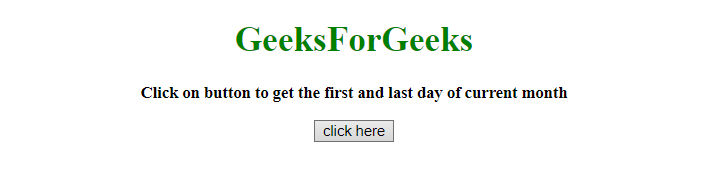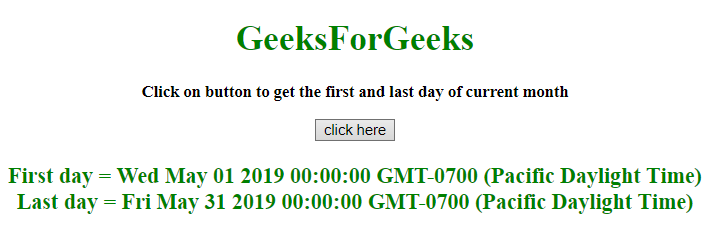# How to get the first and last date of current month using JavaScript ?

Given a month and the task is to determine the first and last day of that month in proper format using JavaScript.

• JavaScript getDate() Method: This method returns the day of the month (from 1 to 31) for the defined date.

Syntax:

`Date.getDate()`

Return value: It returns a number from 1 to 31, representing the day of the month.

• JavaScript getFullYear() Method: This method returns the year (four digits for dates between year 1000 and 9999) of the defined date.

Syntax:

`Date.getFullYear()`

Return value: It returns a number, representing the year of the defined date.

• JavaScript getMonth() Method: This method returns the month (from 0 to 11) for the defined date, based on to local time.

Syntax:

`Date.getMonth()`

Return value: It returns a number, from 0 to 11, representing the month.

Example 1: This example sets the date of the var firstDay and lastDay by getting the year and month of the current date using getFullYear() and getMonth() method and then get the first and last day of the month.

 `  ` `<``html``>  ` `    ``<``head``>  ` `        ``<``title``>  ` `            ``Get first and last date of current month ` `        ``      ` `    ``  ` `     `  `    ``<``body` `style` `= ``"text-align:center;"``>  ` `     `  `        ``<``h1` `style` `= ``"color:green;"` `>  ` `            ``GeeksForGeeks  ` `        `` ` `         `  `        ``<``p` `id` `= ``"GFG_UP"` `style` `=  ` `            ``"font-size: 15px; font-weight: bold;"``> ` `        `` ` `         `  `        ``<``button` `onclick` `= ``"GFG_Fun()"``> ` `            ``click here ` `        `` ` `         `  `        ``<``p` `id` `= ``"GFG_DOWN"` `style` `=  ` `            ``"color:green; font-size: 20px; font-weight: bold;"``> ` `        `` ` `         `  `        ``<``script``> ` `            ``var up = document.getElementById('GFG_UP'); ` `             `  `            ``up.innerHTML = "Click on button to get the first " ` `                    ``+ "and last day of current month"; ` `             `  `            ``var down = document.getElementById('GFG_DOWN');  ` `             `  `            ``function GFG_Fun() { ` `                ``var date = new Date(); ` `                 `  `                ``var firstDay =  ` `                    ``new Date(date.getFullYear(), date.getMonth(), 1); ` `                     `  `                ``var lastDay =  ` `                   ``new Date(date.getFullYear(), date.getMonth() + 1, 0); ` `                    `  `                ``down.innerHTML = "First day = " + firstDay + ` `                            ``"<``br``>Last day = " + lastDay; ` `            ``} ` `        ``  ` `    ``  ` `                     `

Output:

• Before clicking on the button:• After clicking on the button:Example 2: This example is similar to the previous one. This example also sets the date of the var firstDay and lastDay by getting the year and month of the current date using getFullYear() and getMonth() method and then get the first and last day of the month by a different approach.

 `  ` `<``html``>  ` `    ``<``head``>  ` `        ``<``title``>  ` `            ``Get first and last date of current month ` `        ``      ` `    ``  ` `     `  `    ``<``body` `style` `= ``"text-align:center;"``>  ` `     `  `        ``<``h1` `style` `= ``"color:green;"` `>  ` `            ``GeeksForGeeks  ` `        `` ` `         `  `        ``<``p` `id` `= ``"GFG_UP"` `style` `=  ` `            ``"font-size: 15px; font-weight: bold;"``> ` `        `` ` `         `  `        ``<``button` `onclick` `= ``"GFG_Fun()"``> ` `            ``click here ` `        `` ` `         `  `        ``<``p` `id` `= ``"GFG_DOWN"` `style` `=  ` `            ``"color:green; font-size: 20px; font-weight: bold;"``> ` `        `` ` `         `  `        ``<``script``> ` `            ``var up = document.getElementById('GFG_UP'); ` `             `  `            ``up.innerHTML = "Click on button to get the first " ` `                    ``+ "and last day of current month"; ` `             `  `            ``var down = document.getElementById('GFG_DOWN');  ` `             `  `            ``function daysInMonth (month, year) { ` `                ``return new Date(year, month, 0).getDate(); ` `            ``} ` `             `  `            ``function GFG_Fun() { ` `                ``var date = new Date(); ` `                ``var firstDay = new Date(date.getFullYear(), ` `                                ``date.getMonth(), 1); ` `                                 `  `                ``var lastDay = new Date(date.getFullYear(), ` `                        ``date.getMonth(), daysInMonth(date.getMonth()+1, ` `                        ``date.getFullYear())); ` `                         `  `                ``down.innerHTML = "First day = " + firstDay + ` `                            ``"<``br``>Last day = " + lastDay; ` `            ``} ` `        ``  ` `    ``  ` `                     `

Output:

• Before clicking on the button:• After clicking on the button:My Personal Notes arrow_drop_upCheck out this Author's contributed articles.

If you like GeeksforGeeks and would like to contribute, you can also write an article using contribute.geeksforgeeks.org or mail your article to contribute@geeksforgeeks.org. See your article appearing on the GeeksforGeeks main page and help other Geeks.

Please Improve this article if you find anything incorrect by clicking on the "Improve Article" button below.